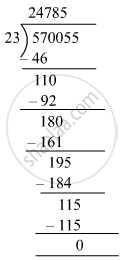# The cost price of 23 colour television sets is Rs 570055. Determine the cost price of each TV set if each costs the same. - Mathematics

Fill in the Blanks

The cost price of 23 colour television sets is Rs 570055. Determine the cost price of each TV set if each costs the same.

#### Solution

Cost price of 23 colour TV sets = Rs 5,70,055
Cost price of 1 TV set = Rs 570055 ÷ 23∴ The cost price of one TV set is Rs 24,785.

Is there an error in this question or solution?

#### APPEARS IN

RS Aggarwal Class 6 Mathematics
Chapter 3 Whole Numbers
Exercise 3E | Q 16 | Page 56